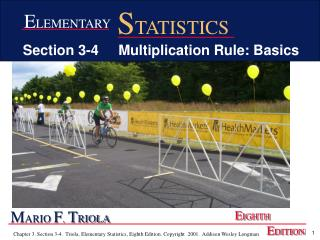DownloadDownload PresentationM ARIO F . T RIOLA

M ARIO F . T RIOLA

Télécharger la présentationM ARIO F . T RIOLA

- - - - - - - - - - - - - - - - - - - - - - - - - - - E N D - - - - - - - - - - - - - - - - - - - - - - - - - - -
Presentation Transcript

1. STATISTICS ELEMENTARY Section 3-4 Multiplication Rule: Basics MARIO F. TRIOLA EIGHTH EDITION

2. Finding the Probability of Two or More Selections Multiple selections means Multiplication Rule

3. Notation P(A and B) = P(event A occurs in a first trial and event B occurs in a second trial)

4. Notation for Conditional Probability P(B A) represents the probability of event B occurring after it is assumed that event A has already occurred (read B A as “B given A”).

5. Definitions • Independent Events Two events A and B are independent if the occurrence of one does not affect the probability of the occurrence of the other. • Dependent Events If A and B are not independent, they are said to be dependent.

6. Formal Multiplication Rule • P(A and B) = P(A) • P(B A) • If A and B are independent events, P(B A) is really the same as P(B)

7. Figure 3-10 Applying the Multiplication Rule P(A or B) Multiplication Rule Are A and B independent ? Yes P(A and B) = P(A) • P(B) No P(A and B) = P(A) • P(B A)

8. Intuitive Multiplication When finding the probability that event A occurs in one trial and B occurs in the next trial, multiply the probability of event A by the probability of event B, but be sure that the probability of event B takes into account the previous occurrence of event A.

9. Examples • A coin is flipped and a die is rolled. Find the probability of getting a head on the coin and a 4 on the die. • Are the independent events? Yes • Solution: • P(Head and 4) = P(Head) * P(4) = 1/2 * 1/6 = 1/12

10. Examples • A bag contains 3 red balls, 2 blue balls and 5 white balls. Balls are selected with replacement in between. • Are the independent events? Yes because of the replacement. • P( Red and Blue)? = P(Red) * P(Blue) = 3/10 * 2/10 = 6/100 = 3/50 =.06 • P( Red and White and Blue)? = P(Red)*P(White)*P(Blue) = 3/10 *5/10* 2/10 = 30/1000 = 3/100 = .03

11. Examples • A bag contains 3 red balls, 2 blue balls and 5 white balls. Balls are selected without replacement in between. • Are the independent events? No because there are less balls each time. • P( Red and White)= = P(Red) * P(White|Red) = 3/10 * 5/9 = 15/90 = 1/6 =.167 • P( Red and White and Blue) = = P(Red)*P(White|Red)*P(Blue|Red & White) = 3/10 *5/9* 2/8 = 30/720 = 1/24 = .0417 • P( Red and Red)= = P(Red and Red) = P(Red)*P(Red|Red) = 3/10 *2/9 = 6/90 = 1/15 = .0667

12. STANDARD DECK OF CARDS • Standard Deck Consists of 52 Cards • 4 Suits: • Hearts(♥), Clubs(♣), Diamonds(♦), Spades(♠) • 2 Colors: 26 of each color • Red: Hearts and Diamonds, Black: Clubs and Spades • 13 Cards in each Suit: • Ace, 2, 3, 4, 5, 6, 7, 8, 9, 10, Jack, Queen and King • 3 Face Cards in each Suit • Jack, Queen and King • 4 of each card • Ex. There are 4 Aces, 4 Kings, etc.

13. Examples • Two cards are selected from a deck without replacement in between. • Are the independent events? No because there are less cards each time. • P( Heart and Club)= = P(Heart) * P(Club|Heart) = 13/52 * 13/51 = 169/2652 =.0637 • P( Ace and Ace)= = P(Ace)*P(Ace|Ace) = 4/52 *3/51 = 12/2652 = .00452

14. Small Samples from Large Populations If a sample size is no more than 5% of the size of the population, treat the selections as being independent (even if the selections are made without replacement, so they are technically dependent).